# Transformer ratings for a power supply

The transformer ratings depend upon the type of filter and rectifier configuration used. The commonly used filter types are inductor and capacitor input filters. However, due to the increased weight and cost considerations, the inductor filter is not very popular. In most power supplies a voltage regulator is used, which provides extra ripple reduction so that an L-C filter is not required. As a result, a capacitive filter is sufficient for a majority of the applications and is very popular among power supply designers.

The current drawn from the transformer secondary varies with the type of rectifier circuit used. The most commonly used single phase circuits are:

• Half Wave (single diode) - HW
• Full Wave Center Tap (two diodes) - FWCT
• Full Wave Bridge (four diodes) - FWB
• Dual Complementary Recfifier

The half wave rectifier is simple and less costly. However, very high current spikes during the capacitive charging interval and the unidirectional DC current in the transformer secondary require a larger transformer core to avoid saturation. Consequently, the half wave rectifier is not very popular and the only time it may be worth considering is for power levels below one watt.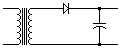Half-Wave

The full wave rectifier circuits are more commonly used. Secondary current surges occur twice per cycle so that they are smaller in magnitude with double the supply frequency. The FWCT rectifier uses two diodes and only half of the transformer secondary is loaded at a time. On the other hand, the FWB rectifier uses 4 diodes and the transformer secondary is loaded continuously. For low voltage supplies, the FWCT is generally preferred while the FWB circuit is more often used for higher voltage supplies.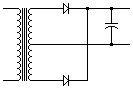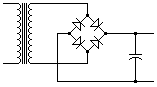Full-Wave or Full-Wave Center Tap Full-Wave Bridge

The Dual Complementary Rectifier Circuit is a combination of two FWCT circuits. This type of rectifier circuit is very commonly used in dual output power supplies, where two outputs are of reversed polarity with a cornmon ground. It is also caued a "center tapped bridge rectifier."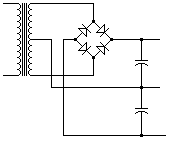Dual Complementary Rectifier Circuit

### Full Wave Center Tap (FWCT)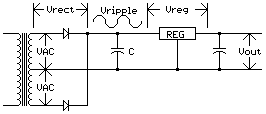The above diagram represents a full wave center-tapped rectifier using capacitive filter. In order to calculate the transformer secondary voltage, following assumptions can be made:

• Vreg, voltage drop across the regulator is approximately 3VDC or more
• Vrect, voltage drop across the rectifier is approximately 1.25VDC or more
• Vripple, the ripple voltage is approximately 10% peak of the output voltage.

Then the following formula can be used to determine the transformer secondary voltage rating: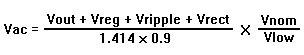where 0.9 is typical rectifier efficiency, Vnom=nominal input line voltage, and Vlow=required low line voltage.

As an example we can consider a power supply with capacitive filter and output of 24VDC at 2.4A to operate at a low line voltage of 95VAC.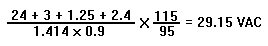Therefore, the transformer secondary voltage may be specified as 59VAC with center tap.

To accurately determine the secondary RMS cuffent rating, one must go through complex calculations. However, for all practical purposes the chart below can be safely used.

Rectifier Type

Rectifier Type Filter Type Secondary RMS Current
Full Wave Center Tap Choke Input = 0.7 x DC Amps
Full Wave Center Tap Capacitor Input = 1 to 1.2 x DC Amps
Full Wave Bridge Choke Input = 1 x DC Amps
Full Wave Center Tap Capacitor Input = 1.6 to 1.8 x DC Amps

In the example above, the transformer secondary RMS current shall be: 1.2 x 2.4 = 2.88 Amps r.m.s. The complete transformer specification can then be defined as: 59VCT @ 2.88 Amps with 170VA

### Full Wave Bridge (FWB)

The same considerations for FWCT also apply to FWB. The only difference is that the rectifier drop is twice as much (4 diodes instead of 2). Therefore, for the same output requirements as above, Vrect = 2 x 1.25 = 2.5V.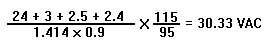That is, VAC can be specified at 30.5VAC. As per the table above, the cuffent rating for capacitive filter shall be 1.8 x 2.4 = 4.32 Amps r.m.s. Thus, the complete transformer specifications can be defined as: 30.5V @ 4.32 Amps with 132VA

### Dual Complementary Supply

The calculation discussed above for FWCT is also applicable to dual complementary circuit. Consider an example of a dual output power supply with 15VDC @ 800mA. Thus, Vout = 15V, Vreg = 3V, Vrect = 1.25V, Vripple = 0.75V (1.0V P-P)

Therefore, VAC = 38 V with center tap; IAC = 1.8 x 800 = 1.44 Amps r.m.s. Thus the transformer secondary rating can be defined as: 38VCT @ 1.44 Amps r.m.s., with 55VA

Finally, a careful attention must be paid to the effect of the high input line. Assuming that the highest input line voltage is 130VAC, the transformer secondary voltage will rise by the ratio 130/115 as compared to the nominal line input. Thus at 130VAC input, for the 24VDC power supply discussed above, VAC = (130/115) x 29.15 = 33.34V

For the dual complemenliry circuit VAC= (130/115) x 19 = 21.5V

The increase in output voltage must be dropped across the regulator, which results in higher power dissipation in the regulator. All specific cases must be checked for safe operation under this condition.

Before final definition of the transformer ratings, load regulation, temperature rise and shielding etc. must also be given due consideration. It must be observed that all the calculated values are at full load rating.